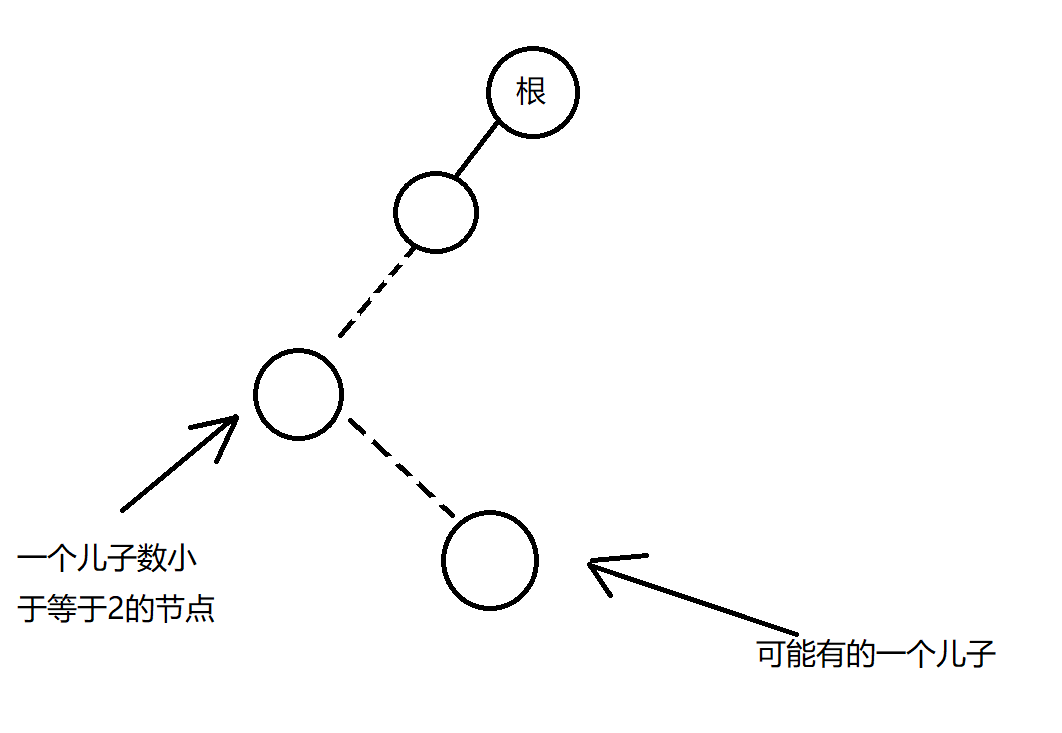# 题解UPD: P.S.图片里的文字是不是写错了？

P.S. 我觉得看题解说分讨不如直接看代码。。。。

# 代码

#include <bits/stdc++.h>
#define clr(x) memset(x,0,sizeof (x))
#define For(i,a,b) for (int i=a;i<=b;i++)
#define Fod(i,b,a) for (int i=b;i>=a;i--)
#define pb(x) push_back(x)
#define mp(x,y) make_pair(x,y)
#define fi first
#define se second
#define _SEED_ ('C'+'L'+'Y'+'A'+'K'+'I'+'O'+'I')
#define outval(x) printf(#x" = %d\n",x)
#define outvec(x) printf("vec "#x" = ");for (auto _v : x)printf("%d ",_v);puts("")
#define outtag(x) puts("----------"#x"----------")
#define outarr(a,L,R) printf(#a"[%d...%d] = ",L,R);\
For(_v2,L,R)printf("%d ",a[_v2]);puts("");
using namespace std;
typedef long long LL;
typedef unsigned long long ULL;
typedef vector <int> vi;
LL read(){
LL x=0,f=0;
char ch=getchar();
while (!isdigit(ch))
f|=ch=='-',ch=getchar();
while (isdigit(ch))
x=(x<<1)+(x<<3)+(ch^48),ch=getchar();
return f?-x:x;
}
const int N=1e6+5;
int n;
vector <int> e[N];
int mi[N];
int son[N];
void dfs(int x,int pre){
mi[x]=e[x].size()!=3?x:n+1;
for (auto y : e[x])
if (y!=pre){
dfs(y,x);
mi[x]=min(mi[x],mi[y]);
son[x][son[x]!=0]=y;
}
if (mi[son[x]]>mi[son[x]])
swap(son[x],son[x]);
}
vector <int> ans;
void calc(int x){
if (!x)
return;
if (son[x]&&son[x])
calc(son[x]),ans.pb(x),calc(son[x]);
else if (x<mi[son[x]])
ans.pb(x),calc(son[x]);
else
calc(son[x]),ans.pb(x);
}
void solve(int x){
ans.pb(x);
if (!son[x])
return;
if (son[x])
calc(son[x]),solve(son[x]);
else {
x=son[x];
if (x<=mi[x])
solve(x);
else
calc(x);
}
}
int main(){
n=read();
For(i,1,n){
int k=read();
while (k--)
e[i].pb(read());
}
int x=mi=n+1;
For(i,1,n)
if (e[i].size()!=3)
x=min(x,i);
dfs(x,0);
solve(x);
for (auto i : ans)
printf("%d ",i);
return 0;
}

posted @ 2019-11-29 10:28  zzd233  阅读(339)  评论(0编辑  收藏  举报